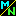Navigation Panel:(These buttons explained below)Simple and Compound Interest

If a bank account produces "simple interest", the interest is paid directly and does not become part of the account's interest-bearing balance. That balance remains constant, and it's easy to figure out how much money you earn. For instance, if a \$100 account earns 10% simple interest a year, the balance remains at \$100 and each year's interest payment is \$10.

The situation is the same for population growth if the offspring produced are not capable of further reproduction: the fertile population, and hence the rate of growth, remain constant.

But what if the offspring can reproduce further? Or, to return to the bank example, what if the interest becomes part of the interest-bearing balance as soon as it is earned, so that you earn interst on the interest, and interest on the interest on the interest, etc. (this is called "compound interest")? How much money will you end up with? How different is it from what you would end up with under simple interest alone?

The answer depends on how large the simple interest is compared to the original amount. If it's only a small fraction, then including it with the original amount won't change that amount by much, so the compound interest (interest on the original amount plus previously earned interest) won't be much different from the simple interest on the original amount alone. The greater the simple interest is compared to the original amount, the more of an effect compounding will have.

Let's ask the question in the case when the ratio is 1 (simple interest = 100% of original amount):

Question: If you would earn 100% interest (i.e., your money would double) under simple interest, how much money would you end up with under compound interest?

This gives us the following physical meaning for the number e:

A Physical Meaning for the Number e

The number e is the factor by which a bank account earning continually compounding interest (or a reproducing population whose offspring are themselves capable of reproduction, or any similar quantity that grows at a rate proportional to its current value) will increase, if, without the compounding (or without the offspring being capable of further reproduction) it would have doubled (increased by 100%).

[Go on]

Navigation Panel: